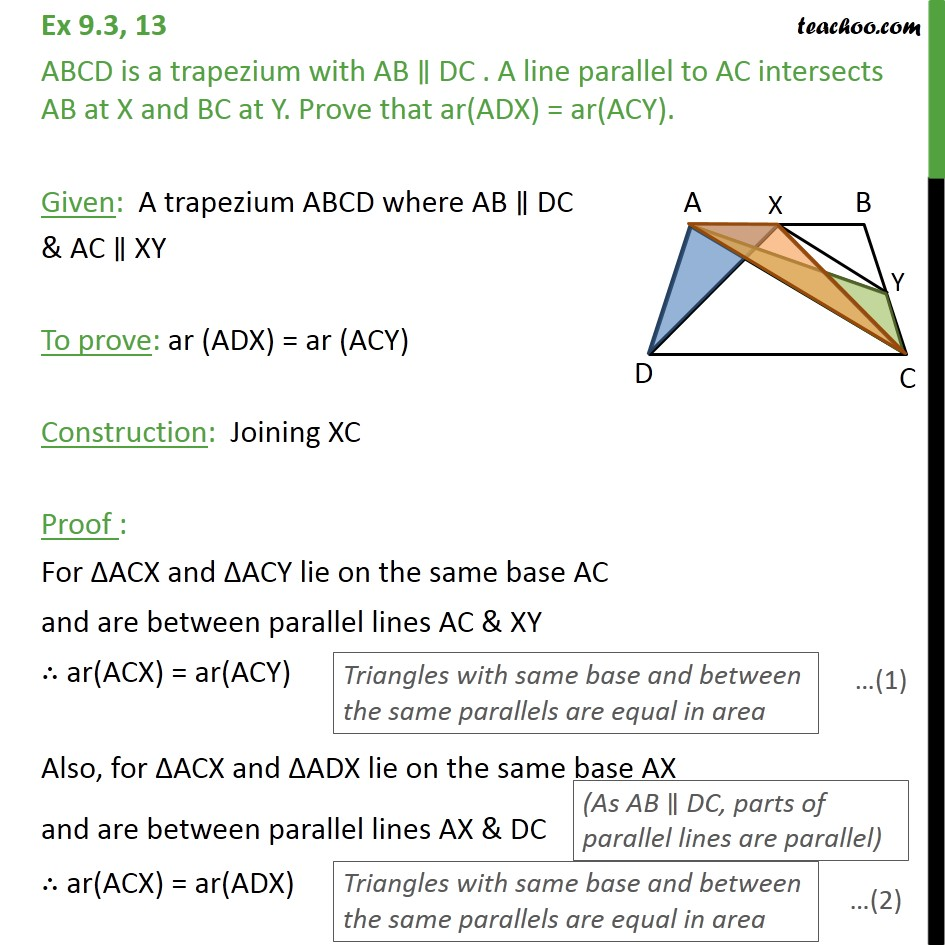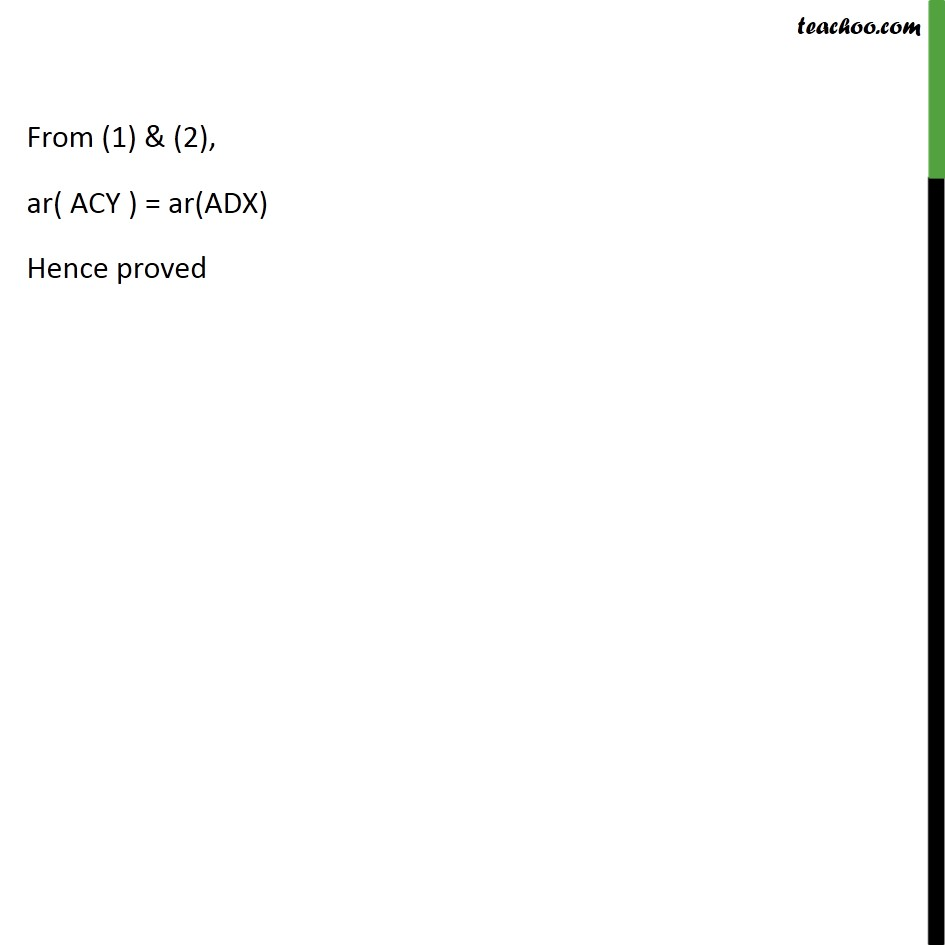Area of Triangles

Areas of Parallelograms and Triangles
Serial order wiseLearn in your speed, with individual attention - Teachoo Maths 1-on-1 Class

### Transcript

Question 13 ABCD is a trapezium with AB ∥ DC . A line parallel to AC intersects AB at X and BC at Y. Prove that ar(ADX) = ar(ACY). Given: A trapezium ABCD where AB ∥ DC & AC ∥ XY To prove: ar (ADX) = ar (ACY) Construction: Joining XC Proof : For ΔACX and ΔACY lie on the same base AC and are between parallel lines AC & XY ∴ ar(ACX) = ar(ACY) Also, for ΔACX and ΔADX lie on the same base AX and are between parallel lines AX & DC ∴ ar(ACX) = ar(ADX) From (1) & (2), ar( ACY ) = ar(ADX) Hence proved